Interactive Image Segmentation

ECSE-626: Statistical Computer Vision

Contents

Introduction

Modeling Segmentation using MRF

Optimization using Graph Cut

Results

Full report, code and data

References

Introduction

Automatic image segmentation is a hard problem and, is not flexible enough to be used for general segmentation. The objective of this project is to implement an interactive image segmentation software based on Yuri Boykov and Marie-Pierre Jolly’s work on “Interactive Graph Cuts for Optimal Boundary & Region Segmentation of Objects in N-D Images” (ICCV 2001) .

Modeling Segmentation using MRF

To solve the problem of segmentation, the problem is first formulated using Markov Random Field (MRF). For this binary segmentation problem, the label set, L is {“obj”, “bkg”} and A is the configuration. The energy function proposed in , is of the following form –Where,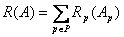,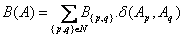and,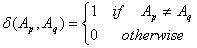Here, R(A) and B(A), which are the likelihood and the prior terms, are known as the regional and boundary term respectively. These terms represent the penalties for labeling a pixel as either object or background. They can be computed as follows –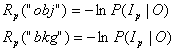and,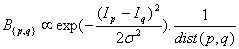The Ip in the above equations can be either pixel values or any other feature vector. The regional penalty is calculated as the negative log-likelihood and therefore if the likelihood of a pixel being of certain class is high the penalty for assigning the pixel to that class is low. The boundary term represents the interaction between two pixels p and q. The exponential component represents the similarity between pixels and the distance component captures the spatial relation between the pixels.

Using this method, the user marks certain regions as either “object” or “background”. The marked pixels are called seeds. These seeds are used as both soft and hard constraints for segmentation. The regional penalty term is computed using these seeds.

Optimization using Graph Cut

The energy function that was defined in the previous section needs to be minimized to solve the segmentation problem. This optimization is done using Graph Cut. For binary segmentation problem, Graph cut can achieve global optimum solution.

The first step in Graph cut optimization is to define the problem as a min-cut problem. This is done by constructing a graph as follows –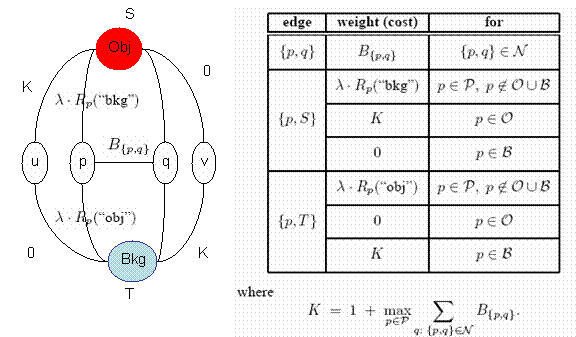Figure 1: Graph Construction

Now, max-flow/min-cut algorithm can be used for finding an optimal cut and thereby an optimal solution to the segmentation problem.

Results

One of the strong points of interactive image segmentation is that it is very general. For instance, this algorithm can be used for doing photo edition (Figure 2) as well as segmenting medical images (Figure 3).  Some of the results obtained using the implementation done as part of this project is provided below.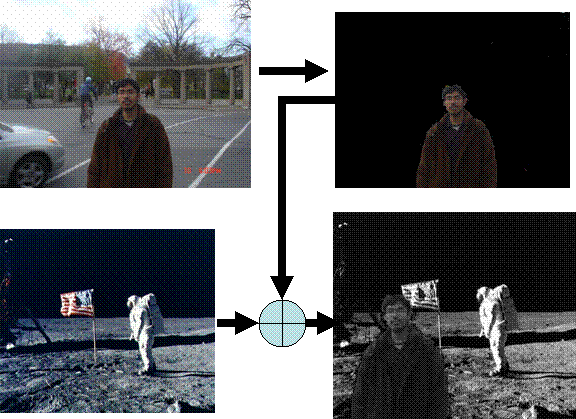Figure 2: Photo Editing using Interactive Image Segmentation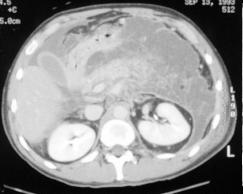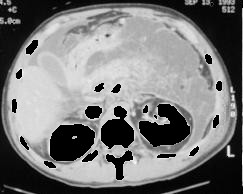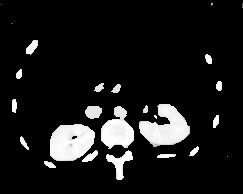Figure 3: Medical Image Segmentation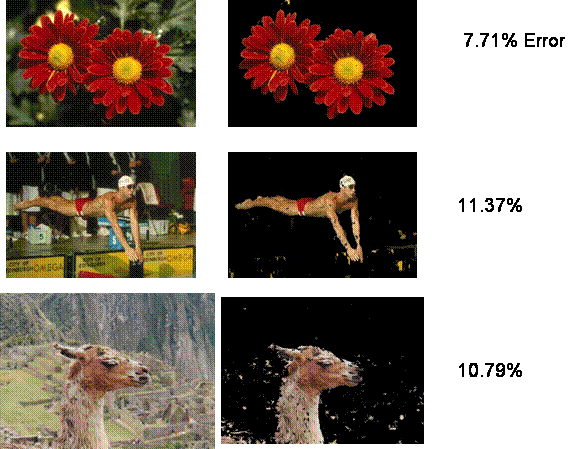Code and Data

Full Report

Code

The implementation uses max-flow/min-cut algorithm developed in .

Benchmark data can be found at   and .

References

 Boykov, Y., Jolly, M.P."Interactive graph cuts for optimal boundary and region segmentation of objects in N-D images". In Proc. Int. Conf. on Computer Vision, vol. 1, pp. 105-112, July 2001.

  Yuri Boykov and Vladimir Kolmogorov,"An Experimental Comparison of Min-Cut/Max-Flow Algorithms for Energy Minimization in Vision.",In IEEE Transactions on Pattern Analysis and Machine Intelligence (PAMI), September 2004